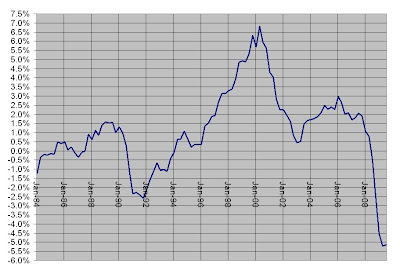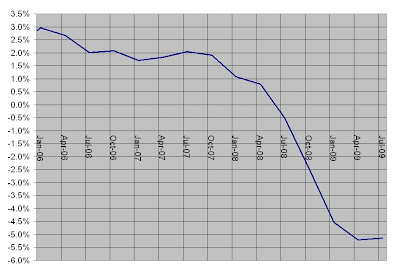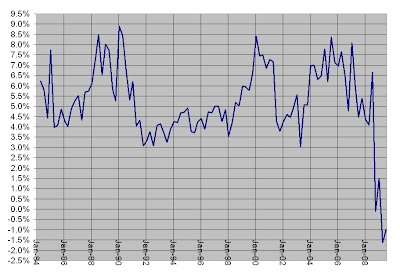## Wednesday, January 20, 2010

### The Taylor Rule

John Taylor wrote:
First of all, I don't think the Taylor Rule does show a large negative interest rates right now. That's kind of a myth. The Taylor Rule is pretty simple. It just says, the interest rate should equal 1 1/2 times the inflation rate, plus 1/2 time the GDP gap, plus 1. Well, if you plug in reasonable estimates for what inflation is and what the GDP gap is, I get a number that’s pretty close to zero. Not minus four, minus five, not numbers like that. So, in fact I would say the amount of quantitative easing could be reduced right now. I hope it is reduced in a gradual way. Some of the mortgage purchases I think could be slowed down and then actually reversed.
That is pretty simple--
R = 1 percent + 1.5 x inflation + .5 x (Real GDP - Potential GDP) /Potential GDP

If the target for the inflation rate is 2 percent and the inflation rate is 2 percent and the economy is producing at capacity, then the proper level for "the" interest rate is 4 percent. If this is expected to persist in the future, then the real interest rate is 2 percent.

If the target for the inflation rate was zero percent (as I prefer) and the economy was producing at capacity, then the target for the interest rate would be 1 percent. If this is expected to persist, the real interest would be 1 percent.

That is a bit of a puzzle, but perhaps the Taylor rule only applies if the target for the inflation rate is two percent.

There are many different measures of inflation. How does the Taylor rule apply using the GDP deflator?

The trend inflation rate during the Great Moderation as measured by the GDP deflator was 2.3 percent. The inflation rate has been well below trend during the Great Recession.Not only is there a question of which inflation rate should be used, the Taylor rule depends on an estimate for potential output. The Federal Reserve bank of St. Louis includes a series for Potential Output sourced from the C.B.O. It uses year 2000 dollars, but that can be easily corrected.The growth rate of this measure of potential output closely tracks the growth rate of Real GDP. The recessions show up as slow or negative growth.

The relationship between the levels of real GDP and this measure of potential output are a bit odd.While Real GDP and this measure of Potential Output track closely during the first decade of the Great Moderation (though with a clear recession in 1991,) real GDP takes off and stays above potential output for most of the remaining period until the Great Recession. The recession of 2001 shows up as an unusual period where Real GDP was close to Potential Output.Focusing on the period of the Great Recession, real GDP was greater than potential output and has now fallen well below potential output. The output gap is the percent difference between the two.This measure of potential income shows very large positive output gaps--unsustainable booms. Focusing in on the Great Recession, the output gap rapidly shifted from positive to negative.Putting together the inflation rate implied by the GDP deflator and the output gap generated by real GDP and the CBO estimate of potential output, the interest rate implied by Taylor's "simple" rule can be calculated.These figures show a "Taylor Rule" interest rate that is less than zero for the last two quarters--1.5 percent and -1 percent. While not -4 percent, they are not zero either. Using these figures to find the interest rates for the entire period shows tremendous volatility.Perhaps the CBO estimate of potential output is off. Or, maybe the GDP deflator is the wrong measure of the price level. However, a third possibility is that trying to find some simple rule that relates the proper interest rate on overnight interbank loans to inflation and the gap between real GDP and some estimate of potential real output is a mistake.

1.The above equation could easily be modified for nominal GDP.

FFR = C1 + C2x(GDPn - Desired path for GDPn)/Desired path for GDPn

You might be interested in the following post
Incorporating NGDP into Taylor rule
http://himaginary.blogspot.com/2009/10/incorporating-ngdp-into-taylor-rule.html

2.Thanks, Bill. Once again, a very enlightening post.

3."Or, maybe the GDP deflator is the wrong measure of the price level."

I think I agree with this. The creation of the electronics industry has resulted in products that massively deflate. This change has increased the variance in the set of time derivatives, of prices at a given time.